#### RFID high frequency coil design principle

Since the wavelength of the electromagnetic wave is far greater than the identification distance, it can be equivalent to a transformer coupling method, and a small loop or square antenna is the best choice.

Antenna equivalent circuit diagram: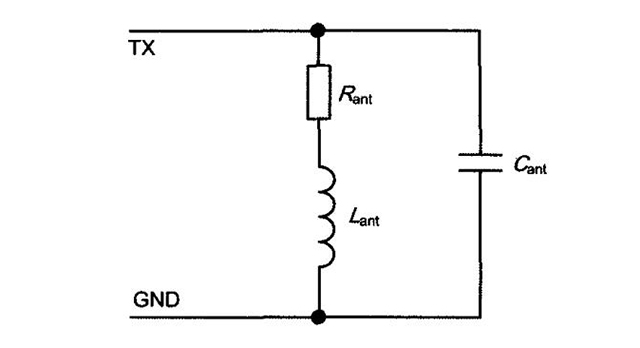Through this resonant circuit, the reader can transmit energy to the radio frequency card and communicate with the card. The resonant frequency can be tuned to the reader’s operating frequency of 13.56MHz. According to the Thomson formula:When the inductance exceeds 5uH, the value of the capacitor is very small. When the inductance is 0.8~1.8uH, the matching of the capacitor is easier to achieve.

However, in actual use, the L value is usually μH, and the C unit is pF. In this case, the fo value can be calculated by the following formula: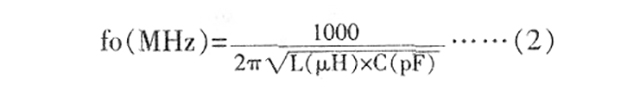Note that at this time, the unit of fo is megahertz (MHz); the unit of L is microhenry (μH); and the unit of C is picofarad (pF). If the C unit is microfarad (μF), the fo unit should be changed to kilohertz (kHz). It can be seen from formula 1 that the product of LC value increases by n times, and fo decreases by N times.

Theoretical basis for antenna size:

The magnetic induction intensity of the magnetic field is proportional to the current Ia flowing through the conductor and inversely proportional to the distance from the conductor.

According to Biosavart’s law, the magnitude of the magnetic induction intensity Bx at a point at a vertical distance x from the center of the ring or square coil is: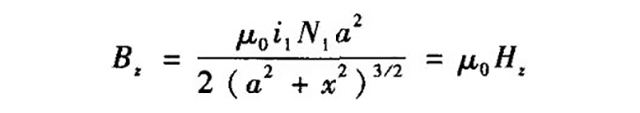In the formula, i is the current; N is the number of turns of the coil; a is the side length of the coil; x is the vertical distance from the center of the coil; u is the vacuum permeability.

The intensity of the magnetic field from the center of the coil to a certain distance is almost constant, and then drops sharply. There is an optimal antenna side length a corresponding to the recognizable distance of each RFID system.

The optimal side length of the reader antenna is equal to √2 times the maximum expected reading distance.

Calculation of antenna inductance value and Q value:

The loop and square antenna inductance can be estimated by the following formula:In the formula, l1 is the length of one turn (turn) of the wire loop (cm); D is the width of the coil wire (mm); K=1.07 (loop antenna) or 1.47 (square antenna); N is the number of turns of the coil; In Is the natural logarithm function.

Assuming that the inductance is 1.6uH, the length of a wire loop is l1=16cm, and the end of the coil is square (K is 1.47), the relationship between the number of coil turns and the line width can be calculated. The line width is generally 0.5~1.5mm.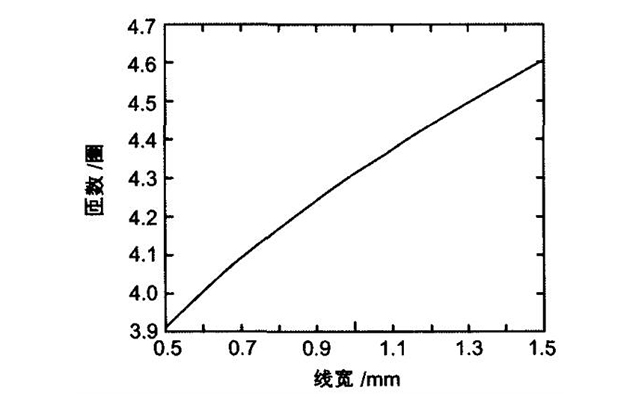The quality factor Q value represents the loss performance of the inductor. The higher the Q value, the higher the output energy of the antenna. However, a too high Q value will interfere with the bandpass characteristics of the reader.

The quality factor of the antenna is defined by the following formula: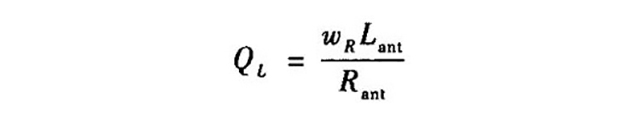In the formula, w=2πf, f is the resonance frequency; R is the equivalent resistance of the antenna; L is the equivalent inductance of the antenna. Generally, the quality factor of RFID system is in the range of 10 to 30, and the maximum should not exceed 60 to ensure sufficient bandwidth. After the compensation coil is added, the magnetic field intensity on the surface of the inductor coil is strengthened. Reason analysis: there is parasitic capacitance in the inductance coil. After adding an open coil at the end, the induced current in the open loop is almost equal to the current in the parasitic capacitance in the inductance coil, but in the opposite direction, which cancels out the parasitic capacitance of the inductance coil. The current is equivalent to avoiding the generation of ground current, reducing the power loss of the inductor coil, thereby relatively increasing the magnetic field strength of the inductor.

عنوان المقر الرئيسي: 3F,Block B,Tongxin Technology Building,No.6261 Baoan Avenue,Fuhai street,Baoan,Shenzhen,Guangdong,P.R.C 518103

الهاتف:+86-755-2699 7700    فاكس:+86-755-2699 7711

Email：info@seaory.com